# Excel: check if two cells match or multiple cells are equal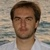by , updated on

The tutorial will teach you how to construct the If match formula in Excel, so it returns logical values, custom text or a value from another cell.

An Excel formula to see if two cells match could be as simple as A1=B1. However, there may be different circumstances when this obvious solution won't work or produce results different from what you expected. In this tutorial, we'll discuss various ways to compare cells in Excel, so you can find an optimal solution for your task.

## How to check if two cells match in Excel

There exist many variations of the Excel If match formula. Just review the examples below and choose the one that works best for your scenario.

### If two cells equal, return TRUE

The simplest "If one cell equals another then true" Excel formula is this:

cell A = cell B

For example, to compare cells in columns A and B in each row, you enter this formula in C2, and then copy it down the column:

`=A2=B2`

As the result, you'll get TRUE if two cells are the same, FALSE otherwise: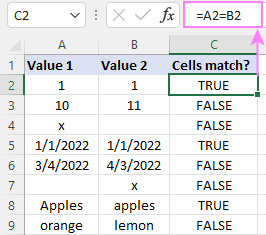Notes:

• This formula returns two Boolean values: if two cells are equal - TRUE; if not equal - FALSE. To only return the TRUE values, use in IF statement as shown in the next example.
• This formula is case-insensitive, so it treats uppercase and lowercase letters as the same characters. If the text case matters, then use this case-sensitive formula.

### If two cells match, return value

To return your own value if two cells match, construct an IF statement using this pattern:

IF(cell A = cell B, value_if_true, value_if_false)

For example, to compare A2 and B2 and return "yes" if they contain the same values, "no" otherwise, the formula is:

`=IF(A2=B2, "yes", "no")`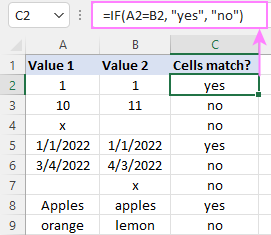If you only want to return a value if cells are equal, then supply an empty string ("") for value_if_false.

If match, then yes:

`=IF(A2=B2, "yes", "")`

If match, then TRUE:

`=IF(A2=B2, TRUE, "")`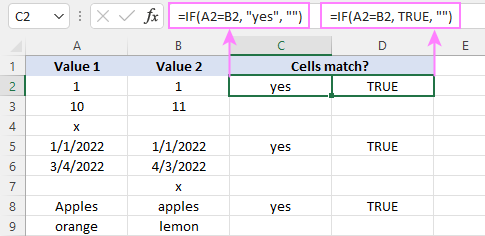Note. To return the logical value TRUE, don't enclose it in double quotes. Using double quotes will convert the logical value into a regular text string.

### If one cell equals another, then return another cell

And here's a variation of the Excel if match formula that solves this specific task: compare the values in two cells and if the data match, then copy a value from another cell.

In the Excel language, it's formulated like this:

IF(cell A = cell B, cell C, "")

For instance, to check the items in columns A and B and return a value from column C if text matches, the formula in D2, copied down, is:

`=IF(A2=B2, C2, "")`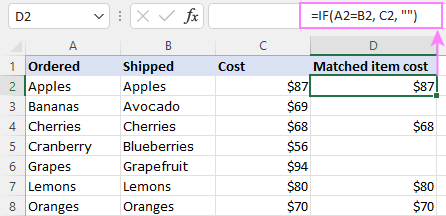### Case-sensitive formula to see if two cells match

In situation when you are dealing with case-sensitive text values, use the EXACT function to compare the cells exactly, including the letter case:

IF(EXACT(cell A, cell B), value_if_true, value_if_false)

For example, to compare the items in A2 and B2 and return "yes" if text matches exactly, "no" if any difference is found, you can use this formula:

`=IF(EXACT(A2, B2), "Yes", "No")`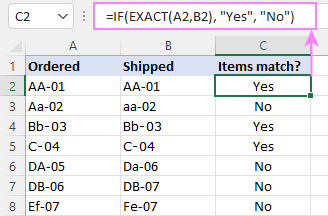## How to check if multiple cells are equal

As with comparing two cells, checking multiple cells for matches can also be done in a few different ways.

### AND formula to see if multiple cells match

To check if multiple values match, you can use the AND function with two or more logical tests:

AND(cell A = cell B, cell A = cell C, …)

For example, to see if cells A2, B2 and C2 are equal, the formula is:

`=AND(A2=B2, A2=C2)`

In dynamic array Excel (365 and 2021) you can also use the below syntax. In Excel 2019 and lower, this will only work as a traditional CSE array formula, completed by pressing the Ctrl + Shift + Enter keys together.

`=AND(A2=B2:C2)`

The result of both AND formulas is the logical values TRUE and FALSE.

To return your own values, wrap AND in the IF function like this:

`=IF(AND(A2=B2:C2), "yes", "")`

This formula returns "yes" if all three cells are equal, a blank cell otherwise.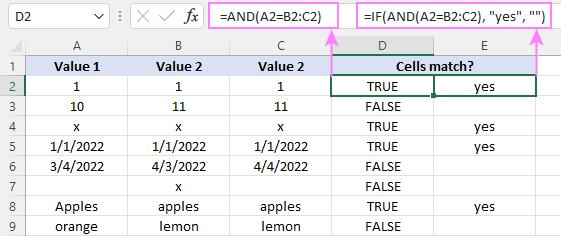### COUNTIF formula to check if multiple columns match

Another way to check for multiple matches is using the COUNTIF function in this form:

COUNTIF(range, cell)=n

Where range is a range of cells to be compared against each other, cell is any single cell in the range, and n is the number of cells in the range.

For our sample dataset, the formula can be written in this form:

`=COUNTIF(A2:C2, A2)=3`

If you are comparing a lot of columns, the COLUMNS function can get the cells' count (n) for you automatically:

`=COUNTIF(A2:C2, A2)=COLUMNS(A2:C2)`

And the IF function will help you return anything you want as an outcome:

`=IF(COUNTIF(A2:C2, A2)=3, "All match", "")`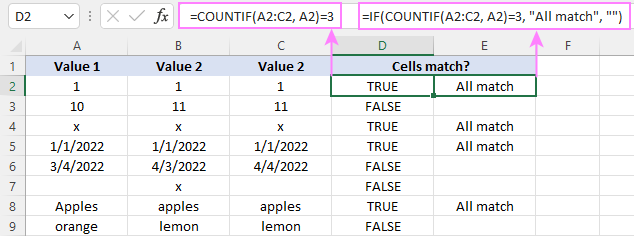### Case-sensitive formula for multiple matches

As with checking two cells, we employ the EXACT function to perform the exact comparison, including the letter case. To handle multiple cells, EXACT is to be nested into the AND function like this:

AND(EXACT(range, cell))

In Excel 365 and Excel 2021, due to support for dynamic arrays, this works as a normal formula. In Excel 2019 and lower, remember to press Ctrl + Shift + Enter to make it an array formula.

For example, to check if cells A2:C2 contain the same values, a case-sensitive formula is:

`=AND(EXACT(A2:C2, A2))`

In combination with IF, it takes this shape:

`=IF(AND(EXACT(A2:C2, A2)), "Yes", "No")`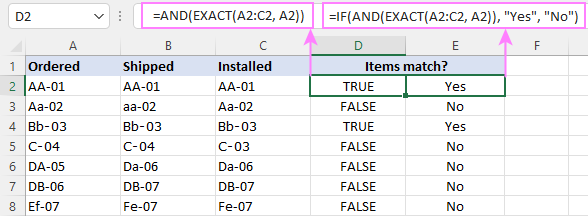## Check if cell matches any cell in range

To see if a cell matches any cell in a given range, utilize one of the following formulas:

OR function

It's best to be used for checking 2 - 3 cells.

OR(cell A = cell B, cell A = cell C, cell A = cell D, …)

Excel 365 and Excel 2021 understand this syntax as well:

OR(cell = range)

In Excel 2019 and lower, this should be entered as an array formula by pressing the Ctrl + Shift + Enter shortcut.

COUNTIF function

COUNTIF(range, cell)>0

For instance, to check if A2 equals any cell in B2:D2, any of these formulas will do:

`=OR(A2=B2, A2=C2, A2=D2)`

`=OR(A2=B2:D2)`

`=COUNTIF(B2:D2, A2)>0`

If you are using Excel 2019 or lower, remember to press Ctrl + Shift + Enter to get the second OR formula to deliver the correct results.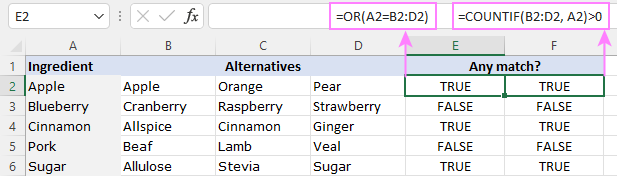To return Yes/No or any other values you want, you know what to do - nest one of the above formulas in the logical test of the IF function. For example:

`=IF(COUNTIF(B2:D2, A2)>0, "Yes", "No")`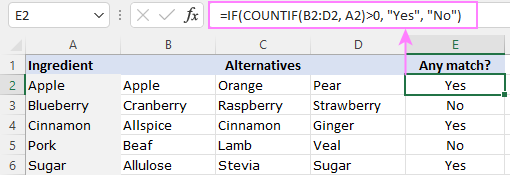## Check if two ranges are equal

To compare two ranges cell-by-cell and return the logical value TRUE if all the cells in the corresponding positions match, supply the equally sized ranges to the logical test of the AND function:

AND(range A = range B)

For example, to compare Matrix A in B3:F6 and Matrix B in B11:F14, the formula is:

`=AND(B3:F6= B11:F14)`

To get Yes/No as the result, use the following IF AND combination:

`=IF(AND(B3:F6=B11:F14), "Yes", "No")`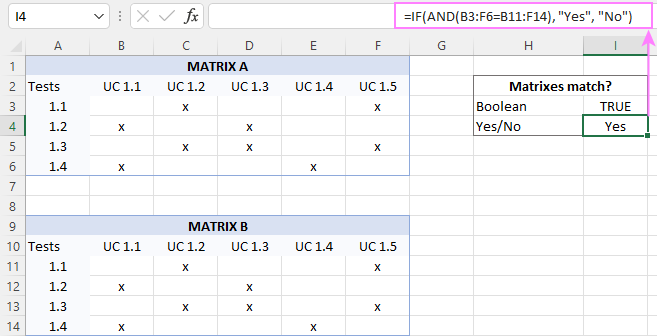That's how to use the If match formula in Excel. I thank you for reading and hope to see you on our blog next week!